# Chapter 1 Real Number NCERT Exemplar Solutions Exercise 1.3 Class 10 Maths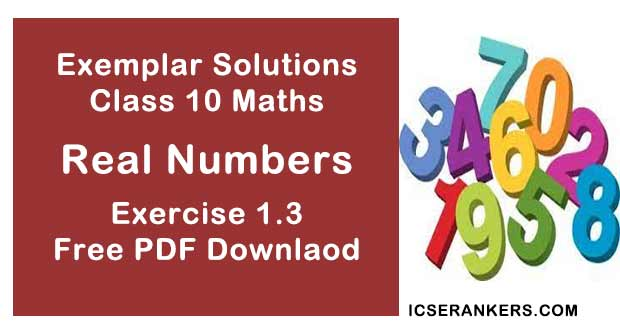Chapter Name NCERT Maths Exemplar Solutions for Chapter 1 Real Numbers Exercise 1.3 Book Name NCERT Exemplar for Class 10 Maths Other Exercises Exercise 1.1Exercise 1.2Exercise 1.4 Related Study NCERT Solutions for Class 10 Maths

### Exercise 1.3 Solutions

1. Show that the square of any positive integer is either of the form 4q or 4q + 1 for some integer q.
Solution
By Euclid’s division lemma,
a = 4m + r ...(i)
Where r = 0 ≤ r < 4
or
r = 0, 1, 2, 3
When r = 0, a = 4m  [From (i)]
When r = 0, we get,
a = 4k
a2 = 16m2
= 4(4m2 )
= 4q, where q = 4m2
When r = 1, we get,
a = 4m + 1
a2 = (4m + 1) 2
= 16m2 + 1 + 8m
= 4(4m + 2)+ 1
= 4q + 1, where q = m(4m + 2)
When r = 2, we get, a = 4m + 2
a2 = (4m + 2) 2
= 16m2 + 4 + 16m
= 4(4m2 + 4m + 1)
= 4q, where q = 4m2 + 4m + 1
When r = 3, we get,
a = 4m + 3
a2 = (4m + 3)2
= 16m2 + 9 + 24m
= 4(4m2 + 6m +2) + 1
= 4q + 1, where q = 4k2 + 6k + 2
Hence, the square of any positive integer is either of the form 4q or 4q + 1 for some integer q.

2. Show that the cube of any positive integer is of the form 4m, 4m + 1 or 4m + 3 for some integer m.
Solution
Let x be any positive integer and b = 4.
Using Euclid Algorithm,
x = bq + r
⇒ x = 4q + r
The possible values of r are,
r = 0,1,2,3
If r = 0,
x = 4q + 0
⇒ x = 4q
Taking cubes on LHS and RHS,
x3 = (4q)3
⇒ x3 = 4(16q3)
x3 = 4m  [where m = 16q3]
If r = 1,
x = 4q + 1
Taking cubes on LHS and RHS,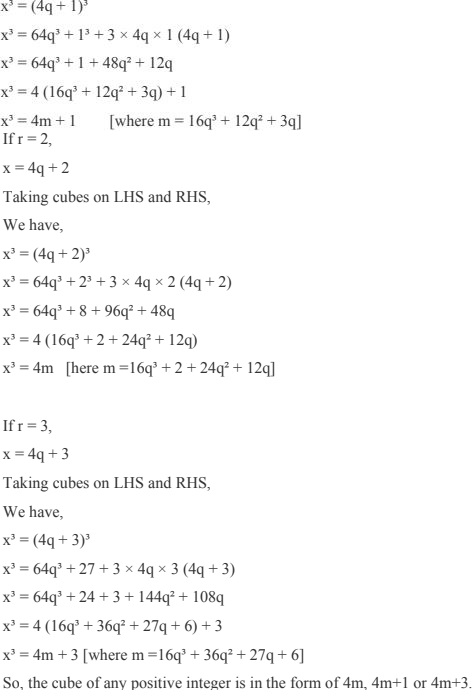3. Show that the square of any positive integer cannot be of the form 5q + 2 or 5q + 3 for any integer q.
Solution
Let the positive integer = x
Using Euclid algorithm,
x = bm + r
According to the question, b = 5
x = 5m + r
So, r = 0, 1, 2, 3, 4
For r = 0, c = 5 m.
For r = 1, x = 5 m + 1
For r = 2, x = 5m + 2.
For r = 3, x = 5m + 3.
For r = 4, x = 5m + 4.
Now, When x = 5m
x2 = (5m)2
= 25m2
x2 = 5(5m2 )
= 5q, where q = 5m2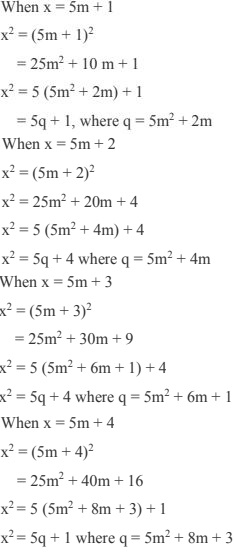So, square of any positive integer cannot be of the form 5q + 2 or 5q + 3.

4. Show that the square of any positive integer cannot be of the form 6m + 2 or 6m + 5 for any integer m.
Solution
Let the positive integer = x
Using Euclid’s algorithm,
x = 6q + r, where 0 ≤ r < 6
x2 = (6q + r)2
= 36q2 + r2 + 12qr
x2 = 6(6q2 + 2qr) + r2
Here, 0 < r < 6
If r = 0, we get
x2 = 6(6q2 )
= 6m,
(Here, m = 6q2 ).
When r = 1, we get
x2 = 6(6q2  + 2q) + 1
= 6m + 1,
Here, m = (6q2 + 2q)
If r = 2, we get
x2 = 6(6q2  + 4q) + 4
= 6m + 4,
Here, m = (6q2  + 4q).
If r = 3, we get
x2 = 6(6q2 + 6q) + 9
= 6(6q2  + 6a) + 6 + 3
x2 = 6(6q2 + 6q + 1) + 3
= 6m + 3,
Here, m = (6q + 6q + 1)
If r = 4, we get
x2 = 6(6q2  + 8q) + 16
= 6(6q2 + 8q) + 12 + 4
x2  = 6(6q2 + 8q + 2) + 4
= 6m + 4,
Here, m = (6q2  + 8q + 2)
If r = 5, we get
x2 = 6(6q2  + 10q) + 25
= 6(6q2  + 10q) + 24 + 1
x2  = 6(6q2  + 10q + 4) + 1
= 6m + 1,
Here, m = (6q2  + 10q + 1).
So, the square of any positive integer cannot be of the form 6m + 2 or 6m + 5 for any integer m.

5. Show that the square of any odd integer is of the form 4q + 1, for some integer q.
Solution
Let c be any odd integer and d = 4.
Using Euclid’s algorithm,
c = 4m + r
and
r = 0,1,2,3 as, 0 ≤ r < 4.
Therefore,
c = 4m, 4m + 1, 4m + 2, 4m + 3
So, c can be 4m + 1 or 4m + 3
c cannot be 4m or 4m + 2, as they are even integer
Now,
(4m + 1)2  = 16m2  + 8m + 1
= 4(4m2  + 2m) + 1
= 4q + 1, where q = 4m2  + 2m.
(4m + 3)2  = 16m2  + 24m + 9
= 4(4m2  + 6m + 2) + 1
= 4q + 1, where q = 4m2  + 6m + 2
Thus we can conclude that square of any odd integer is of the form 4q + 1, for some integer q.

6. If n is an odd integer, then show that n2  – 1 is divisible by 8.
Solution
Let
x = n2 - 1
As n is odd number so,
n = 1, 3, 5, 7
If n = 1
x = 12 - 1
= 0, which is divisible by 8.
If n = 3
x = 32 - 1
= 9 -1
= 8, which is also divisible by 8.
If n = 5,
x = 52 - 1
= 25 - 1
= 24
= 8 × 3, which is divisible by 8.
Therefore, n2 - 1 is divisible by 8 when n is odd.

7. Prove that, if x and y, both are odd positive integers, then (x2 + y2 ) is even but not divisible by 4.
Solution
Let the two odd positive numbers x and y be 2m + 1 and 2n + 1 respectively.
So,
x2 + y2 = (2m + 1)2  + (2n + 1)2
= 4m2  + 4m + 1 + 4n2 + 4n + 1
= 4m2 + 4n2 + 4m + 4n + 2
= 4(m2 + n2 + m + n) + 2
In the above expression we see that the sum of square is even, but the number is not divisible
by 4. Therefore, we can say that if x and y are odd positive integer, then x2 + y2  is even but not divisible by four.

8. Use Euclid’s division algorithm to find HCF of 441, 567 and 693.
Solution
Let p = 693 and q = 567
Using Euclid's division lemma,
p = qm + r
693 = 567 × 1 + 126
567 = 126 × 4 + 63
126 = 63 × 2 + 0
So, HCF (693 and 567) = 63.
Further,
Taking 441 and HCF = 63
Using Euclid’s algorithm,
c = dm + r
⇒ c = 441 and d = 63
⇒ 441 = 63 × 7 + 0
So, HCF of (693, 567, 441) = 63.

9. Using Euclid’s division algorithm, find the largest number that divides 1251, 9377 and 1562 8 leaving remainders, 1, 2, and 3 respectively.
Solution
According to question 1, 2, and 3 are the remainders when the largest number divides 1251,
9377 and 15628 respectively.
So, we have to find HCF of (1251 – 1), (9377 – 2) and (15628 – 3)
That are,
1250, 9375, 15625
For HCF of 1250, 9375, 15625
Let p = 15625,
q = 9375
Using Euclid’s algorithm, p = qm+ r
15625 = 9375 × 1 + 6250
9375 = 6250 × 1 + 3125
6250 = 3125 × 2 + 0
Therefore,
HCF (15625, 9375) = 3125
Let a = 1250 and
b = 3125
By Euclid’s division algorithm, b = am + r
3125 = 1250 × 2 + 625
1250 = 625 × 2 + 0
Therefore, the HCF of (15625, 1250 and 9375) is 625.

10. Prove that (√3 + √5) is irrational.
Solution
Let us consider (√3 + √5) is a rational number that can be written as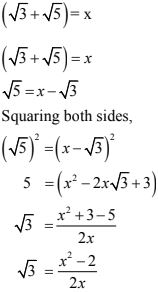From the above expression, RHS comes out to be rational but we know that LHS = √3 is irrational, which contradicts our fact. So, (√3 + √5) is irrational.

11. Show that 12n cannot end with the digit 0 or 5 for any natural number n.
Solution
Number ending at 0 or 5 must be divisible by 5.
So,
(12)n  = (2 × 2 × 3)n
= 2n × 3n
(12)n  does not have 5 as its factor. So, 12n   can never end with 5 and zero.

12. On a morning walk, three persons, step off together and their steps measure 40cm,42 cm and 45cm respectively. What is the minimum distance each should walk, so that each can cover the same distance in complete steps?
Solution
Then minimum distance = LCM of covered steps.
40 = 23 × 5
42 = 2 × 3 ×7
45 = 32 × 5
LCM of (40, 42, 45) = 23 × 32 × 5 × 7 = 2520 cm
Hence, the minimum distance each should walk is 2520 cm.

13. Write the denominator of rational number 257/5000 in the form of 2m × 5n , where m, n are non-negative integers. Hence, write its decimal expansion, without actual division.
Solution
We have, denominator of the rational number 27/5000 = 5000
Therefore,
5000 = 23 × 54
It is of the form 2m × 5n
Here m = 3 and n =  4
So,
257/5000 = (257 × 2)/(5000 × 2) = 0.0514

14. Prove that (√p + √q) is irrational, where p and q are primes.
Solution
Let us take (√p + √q) = rational and can be represented as (√P + √q) = x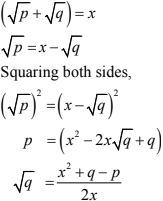As q is prime so √q is no rational but in above solution we had √q = rational because a, p, q are non - zero integers which contradicts our fact.
So, (√p + √q) is irrational.